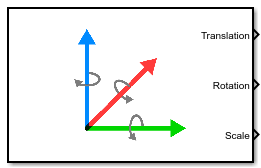# Simulation 3D Actor Transform Get

Get actor translation, rotation, scale

• Library:
• Vehicle Dynamics Blockset / Vehicle Scenarios / Sim3D / Sim3D Core

Aerospace Blockset / Animation / Simulation 3D

•## Description

The Simulation 3D Actor Transform Get block provides the actor translation, rotation, and scale for the Simulink® simulation environment.

The block uses a vehicle-fixed coordinate system that is initially aligned with the inertial world coordinate system.AxisDescription
X

Forward direction of the vehicle

Roll — Right-handed rotation about X-axis

Y

Extends to the right of the vehicle, initially parallel to the ground plane

Pitch — Right-handed rotation about Y-axis

Z

Extends upwards

Yaw — Left-handed rotation about Z-axis

Actors are scene objects that support 3D translation, rotation, and scale. Parts are actor components. Components do not exist by themselves; they are associated with an actor.

Tip

Verify that the Simulation 3D Scene Configuration block executes before the Simulation 3D Actor Transform Get block. That way, the Unreal Engine® 3D visualization environment prepares the data before the Simulation 3D Actor Transform Get block receives it. To check the block execution order, right-click the blocks and select Properties. On the General tab, confirm these Priority settings:

• Simulation 3D Scene Configuration`0`

• Simulation 3D Actor Transform Get`1`

## Ports

### Output

expand all

Actor translation, in m. Array dimensions are number of parts per actor-by-`3`.

• `Translation(1,1)`, `Translation(1,2)`, and `Translation(1,3)` — Vehicle displacement along world X-, Y, and Z- axes, respectively.

• `Translation(...,1)`, `Translation(...,2)`, and `Translation(...,3)` — Actor displacement relative to vehicle, in vehicle-fixed coordinate system initially aligned with world X-, Y, and Z- axes, respectively.

For example, consider a vehicle actor with a vehicle body and four wheels. The `Translation` signal:

• Dimensions are `[5x3]`.

• Contains translation information according to the axle and wheel locations, relative to vehicle.

`$Translation=\left[\begin{array}{ccc}{X}_{v}& {Y}_{v}& {Z}_{v}\\ {X}_{FL}& {Y}_{FL}& {Z}_{FL}\\ {X}_{FR}& {Y}_{FR}& {Z}_{FR}\\ {X}_{RL}& {Y}_{RL}& {Z}_{RL}\\ {X}_{RR}& {Y}_{RR}& {Z}_{RR}\end{array}\right]$`

TranslationArray Element

Vehicle, Xv

`Translation(1,1)`

Vehicle, Yv

`Translation(1,2)`

Vehicle, Zv

`Translation(1,3)`

Front left wheel, XFL

`Translation(2,1)`

Front left wheel, YFL

`Translation(2,2)`

Front left wheel, ZFL

`Translation(2,3)`

Front right wheel, XFR

`Translation(3,1)`

Front right wheel, YFR

`Translation(3,2)`

Front right wheel, ZFR

`Translation(3,3)`

Rear left wheel, XRL

`Translation(4,1)`

Rear left wheel, YRL

`Translation(4,2)`

Rear left wheel, ZRL

`Translation(4,3)`

Rear right wheel, XRR

`Translation(5,1)`

Rear right wheel, YRR

`Translation(5,2)`

Rear right wheel, ZRR

`Translation(5,3)`

Actor rotation across a [-pi/2, pi/2] range, in rad. Array dimensions are number of parts per actor-by-`3`.

• `Rotation(1,1)`, `Rotation(1,2)`, and `Rotation(1,3)` — Vehicle rotation about vehicle-fixed pitch, roll, and yaw Y-, Z-, and X- axes, respectively.

• `Rotation(...,1)`, `Rotation(...,2)`, and `Rotation(...,3)` — Actor rotation about vehicle-fixed pitch, roll, and yaw Y-, Z-, and X- axes, respectively.

For example, consider a vehicle actor with a vehicle body and four wheels. The `Rotation` signal:

• Dimensions are `[5x3]`.

• Contains rotation information according to the axle and wheel locations.

`$Rotation=\left[\begin{array}{ccc}Pitc{h}_{v}& Rol{l}_{v}& Ya{w}_{v}\\ Pitc{h}_{FL}& Rol{l}_{FL}& Ya{w}_{FL}\\ Pitc{h}_{FR}& Rol{l}_{FR}& Ya{w}_{FR}\\ Pitc{h}_{RL}& Rol{l}_{RL}& Ya{w}_{RL}\\ Pitc{h}_{RR}& Rol{l}_{RR}& Ya{w}_{RR}\end{array}\right]$`

RotationArray Element

Vehicle, Pitchv

`Rotation(1,1)`

Vehicle, Rollv

`Rotation(1,2)`

Vehicle, Yawv

`Rotation(1,3)`

Front left wheel, PitchFL

`Rotation(2,1)`

Front left wheel, RollFL

`Rotation(2,2)`

Front left wheel, YawFL

`Rotation(2,3)`

Front right wheel, PitchFR

`Rotation(3,1)`

Front right wheel, RollFR

`Rotation(3,2)`

Front right wheel, YawFR

`Rotation(3,3)`

Rear left wheel, PitchRL

`Rotation(4,1)`

Rear left wheel, RollRL

`Rotation(4,2)`

Rear left wheel, YawRL

`Rotation(4,3)`

Rear right wheel, PitchRR

`Rotation(5,1)`

Rear right wheel, RollRR

`Rotation(5,2)`

Rear right wheel, YawRR

`Rotation(5,3)`

Actor scale. Array dimensions are number of number of parts per actor-by-`3`.

• `Scale(1,1)`, `Scale(1,2)`, and `Scale(1,3)` — Vehicle scale along world X-, Y-, and Z- axes, respectively.

• `Scale(...,1)`, `Scale(...,2)`, and `Scale(...,3)` — Actor scale along world X-, Y-, and Z- axes, respectively.

For example, consider a vehicle actor with a vehicle body and four wheels. The `Scale` signal:

• Dimensions are `[5x3]`.

• Contains scale information according to the axle and wheel locations.

`$Scale=\left[\begin{array}{ccc}{X}_{{V}_{scale}}& {Y}_{{V}_{scale}}& {Z}_{{V}_{scale}}\\ {X}_{F{L}_{scale}}& {Y}_{F{L}_{scale}}& {Z}_{F{L}_{scale}}\\ {X}_{F{R}_{scale}}& {Y}_{F{R}_{scale}}& {Z}_{F{R}_{scale}}\\ {X}_{R{L}_{scale}}& {Y}_{R{L}_{scale}}& {Z}_{R{L}_{scale}}\\ {X}_{R{R}_{scale}}& {Y}_{R{R}_{scale}}& {Z}_{R{R}_{scale}}\end{array}\right]$`

ScaleArray Element

Vehicle, Xvscale

`Scale(1,1)`

Vehicle, Yvscale

`Scale(1,2)`

Vehicle, Zvscale

`Scale(1,3)`

Front left wheel, XFLscale

`Scale(2,1)`

Front left wheel, YFLscale

`Scale(2,2)`

Front left wheel, ZFLscale

`Scale(2,3)`

Front right wheel, XFRscale

`Scale(3,1)`

Front right wheel, YFRscale

`Scale(3,2)`

Front right wheel, ZFRscale

`Scale(3,3)`

Rear left wheel, XRLscale

`Scale(4,1)`

Rear left wheel, YRLscale

`Scale(4,2)`

Rear left wheel, ZRLscale

`Scale(4,3)`

Rear right wheel, XRRscale

`Scale(5,1)`

Rear right wheel, YRRscale

`Scale(5,2)`

Rear right wheel, ZRRscale

`Scale(5,3)`

## Parameters

expand all

Actor name.

Actors are scene objects that support 3D translation, rotation, and scale. Parts are actor components. Components do not exist by themselves; they are associated with an actor.

The block does not support multiple instances of the same actor tag. To refer to the same scene actor when you use the 3D block pairs (e.g. Simulation 3D Actor Transform Get and Simulation 3D Actor Transform Set), specify the same Tag for actor in 3D scene, ActorTag parameter.

Number of parts per actor. Actors are scene objects that support 3D translation, rotation, and scale. Parts are actor components. Components do not exist by themselves; they are associated with an actor. Typically, a vehicle actor with a body and four wheels has 5 parts.

The block does not support multiple instances of the same actor tag. To refer to the same scene actor when you use the 3D block pairs (e.g. Simulation 3D Actor Transform Get and Simulation 3D Actor Transform Set), specify the same Tag for actor in 3D scene, ActorTag parameter.

Sample time, Ts. The graphics frame rate is the inverse of the sample time.

## Version History

Introduced in R2021b